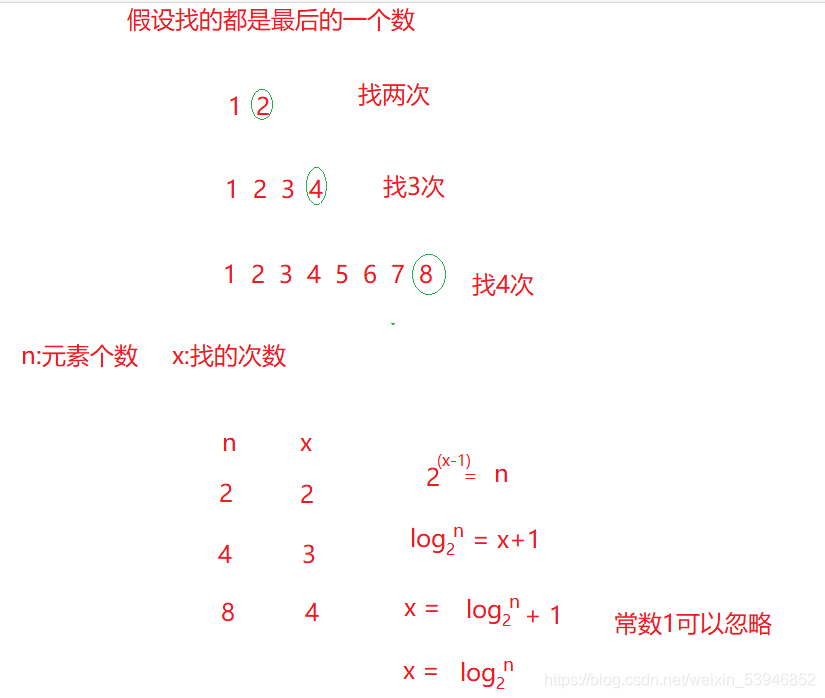﻿ Java算法之时间复杂度和空间复杂度的概念和计算_java_脚本之家
java# Java算法之时间复杂度和空间复杂度的概念和计算

## 二、时间复杂度

### 2.2 大O的渐进表示法

(1)推导大O阶方法

``` void func(int N){
int count = 0;//执行1次
for (int i = 0; i < N ; i++) {//执行N*N次
for (int j = 0; j < N ; j++) {
count++;
}
}
for (int k = 0; k < 2 * N ; k++) {//执行2*N次
count++;
}
int M = 10;//执行1次
while ((M--) > 0) {//执行10次
count++;
}
System.out.println(count);
}
```

在修改后的运行次数函数中，只保留最高阶项。

如果最高阶项存在且不是1，则去除与这个项目相乘的常数。得到的结果就是大O阶

(1) 最坏情况

(2)最好情况

(3)平均情况

### 2.4 常见时间复杂度计算举例

#### 2.4.1 例子

```void func2(int N) {
int count = 0;//1
for (int k = 0; k < 2 * N ; k++) { //2*N
count++;
}
int M = 10;//1
while ((M--) > 0) {//10
count++;
}
System.out.println(count);
}
```

1+2*N+1+10 通过推导大O阶方法后:时间复杂度为 O(N)

```void func3(int N, int M) {
int count = 0;//常数可以不加
for (int k = 0; k < M; k++) {//M
count++;
}
for (int k = 0; k < N ; k++) {//N
count++;
}
System.out.println(count);
}
```

```void func4(int N) {
int count = 0;
for (int k = 0; k < 100; k++) {//用常数1取代运行时间中的所有加法常数
count++;
}
System.out.println(count);
}```

#### 2.4.2 冒泡排序时间复杂度

```void bubbleSort(int[] array) {
for (int end = array.length; end > 0; end--) {
boolean sorted = true;
for (int i = 1; i < end; i++) {
if(array[i - 1] > array[i]){
Swap(array, i - 1, i);
sorted = false;
}
}
if (sorted == true) {
break;
}
}
}
```

#### 2.4.3 二分查找的时间复杂度

```public static int binarySearch(int[] arr,int x){
int left = 0;
int right = arr.length-1;
int mid = 0;//中间下标

while(left <= right){
mid = left+(right-left)/2;
if(arr[mid] > x){
right = mid - 1;
}else if(arr[mid] < x){
left = mid+1;
}else{
return mid;
}
}

return -1;
}
```#### 2.4.4 递归的时间复杂度

```long factorial(int N) {
return N < 2 ? N : factorial(N-1) * N;
}```

```public static int fib(int n){
if(n==1||n==2){
return 1;
}else{
return fib(n-1)+fib(n-2);
}
}```## 三、空间复杂度

### 3.2 空间复杂度的计算

(1) 冒泡排序

```void bubbleSort(int[] array) {
for (int end = array.length; end > 0; end--) {
boolean sorted = true;//额外变量
for (int i = 1; i < end; i++) {
if (array[i - 1] > array[i]) {
Swap(array, i - 1, i);
sorted = false;
}
}
if (sorted == true) {
break;
}
}
}
```

(2) 斐波那契数列

```public static long[] fibonacci(int n) {
long[] fibArray = new long[n + 1];//额外空间
fibArray = 0;
fibArray = 1;
for (int i = 2; i <= n ; i++) {
fibArray[i] = fibArray[i - 1] + fibArray [i - 2];
}
return fibArray;
}
```

(3)递归

```long factorial(int N) {
return N < 2 ? N : factorial(N-1)*N;
}```

## 四、总结

1.在平常我们所说的时间复杂度一般说的都是算法的最坏情况
2.时间复杂度度是一个函数，这个函数只能大致估一下这个算法的时间复杂度
3.空间复杂度是个算法在运行过程中额外占用存储空间大小的量度